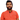# Learn Pain LessHomeOur TeamContact
Python
How to Add Correlation Coefficient to Scatter Plot in PythonPawneshwer Gupta
March 12, 2023
1 min

01
Step 1: Import the necessary libraries
02
Step 2: Create the data
03
Step 3: Plot the scatter plot
04
Step 4: Add the correlation coefficient
05
Step 5: Display the plotAs a business owner or data analyst, you may need to analyze the correlation between two variables. The correlation coefficient is a statistical measure that helps you determine the degree of correlation between two variables. In Python, you can easily plot a scatter plot to visualize the relationship between two variables. However, you may also need to add the correlation coefficient to the plot to get a better understanding of the relationship. In this article, we will show you how to add a correlation coefficient to a scatter plot in Python.

## .css-1qh5hbx{box-sizing:border-box;margin:0;min-width:0;display:block;color:var(--theme-ui-colors-heading,#2d3748);font-weight:bold;-webkit-text-decoration:none;text-decoration:none;margin-bottom:1rem;font-size:1.5rem;position:relative;}Step 1: Import the necessary libraries

To plot the scatter plot and add the correlation coefficient, you will need to import the following libraries:

`.css-1baulvz{display:inline-block;}import matplotlib.pyplot as pltimport numpy as npimport scipy.stats as stats`

## Step 2: Create the data

Next, you will need to create the data that you want to plot. In this example, we will create two arrays of random data using the NumPy library.

`x = np.random.randn(100)y = np.random.randn(100)`

## Step 3: Plot the scatter plot

Now, you can plot the scatter plot using the following code:

`plt.scatter(x, y)plt.title('Scatter plot with correlation coefficient')plt.xlabel('X')plt.ylabel('Y')`

This will create a basic scatter plot that shows the relationship between the two variables.

## Step 4: Add the correlation coefficient

To add the correlation coefficient to the scatter plot, you will need to use the `stats.pearsonr` function from the SciPy library. This function calculates the Pearson correlation coefficient and the p-value for testing non-correlation.

`r, p = stats.pearsonr(x, y)plt.annotate('r = {:.2f}'.format(r), xy=(0.7, 0.9), xycoords='axes fraction')`

This code will add the correlation coefficient (r) to the scatter plot in the top right corner. The `xy` the argument specifies the position of the text, and `xycoords` specifies the coordinate system.

## Step 5: Display the plot

Finally, you can display the plot using the `plt.show()` function.

`plt.show()`

Congratulations, you have now successfully plotted a scatter plot with the correlation coefficient in Python!

In conclusion, adding the correlation coefficient to a scatter plot in Python is an essential step in data analysis. By visualizing the relationship between two variables and adding the correlation coefficient, you can gain valuable insights into your data. We hope this article has been helpful in showing you how to add the correlation coefficient to a scatter plot in Python.

We'll send you the best of our blog just once a month. We promise.

QuestionHow To

## Software Developer

Pawneshwer Gupta works as a software engineer who is enthusiastic in creating efficient and innovative software solutions.

Python
Flutter
Laravel
NodeJS

## Related PostsHow to understand modulus % in python
March 28, 2023
1 min